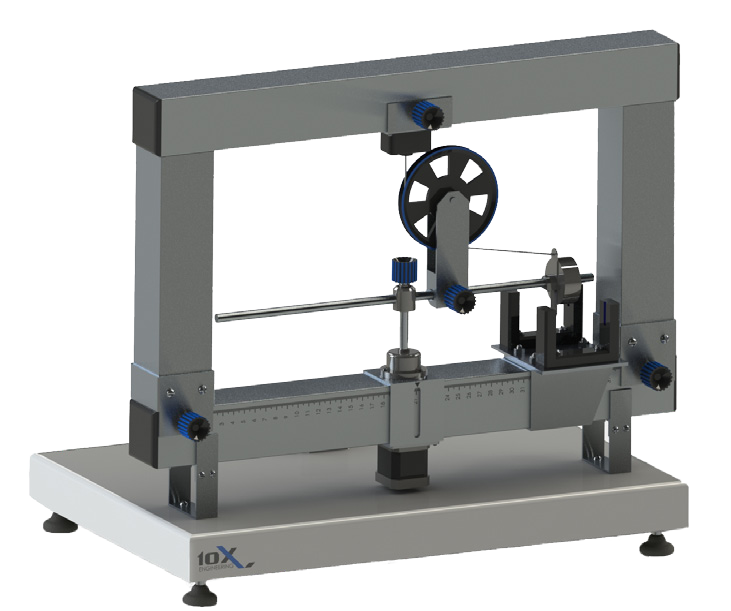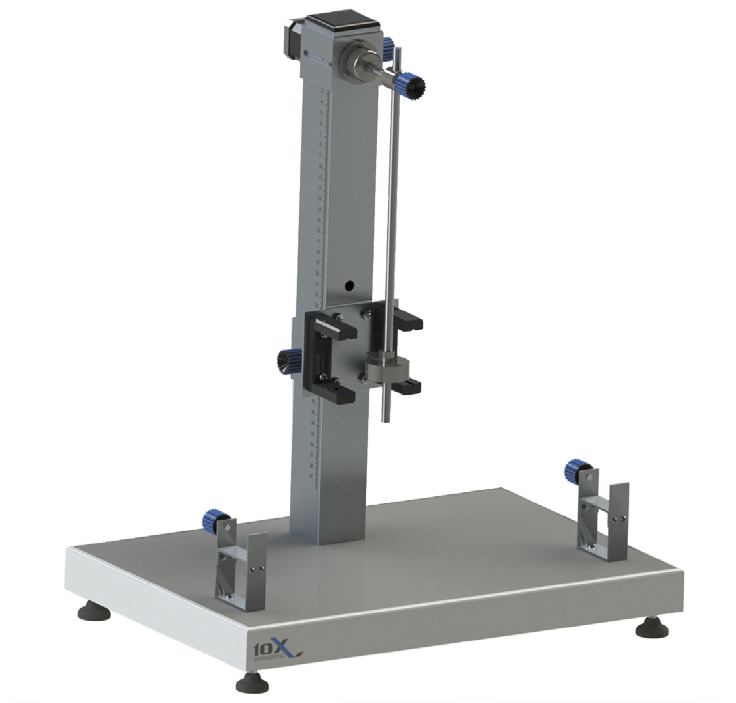### Mechanical Oscillations and Circular Motion Lab

Contribution of current laboratory facility for hands on study of principles of mechanical vibration and circular motion improves the quality and efficiency of studying procedure. The laboratory stand transformation and software flexibility allows student to use a single stand for both vibration and circular motions investigations. The software contains all necessary theoretical materials for laboratory work with graphical measurement representation. Right proportional combination of mechanical and automated procedures, experiments results calculations makes this lab-stand ideal tool for interactive and interesting experiments and research works.

List of labs
• Oscillations, calculation of the period and frequency by using software timers
• Spring pendulum, determination of elastic constant k, harmonic oscillations in a spring pendulum
• Harmonic oscillations in an ideal mathematical pendulum
• Determination of free-fall acceleration using a Pendulum
• Energy transformations during mechanical oscillations
• Determination of the tangential component of acceleration
• Angular velocity of Circular motion
• Determination of centripetal acceleration
• Determination of the centrifugal force depending on the mass
• Determination of the centrifugal force depending on the radius
• Determination of the centrifugal force depending on the angular speed